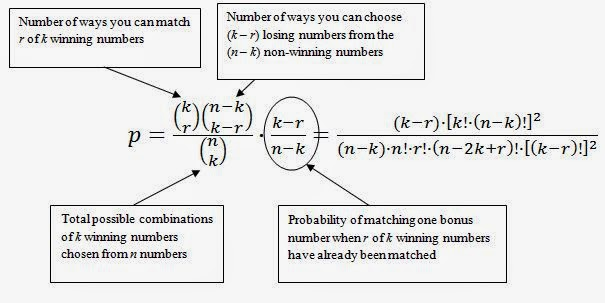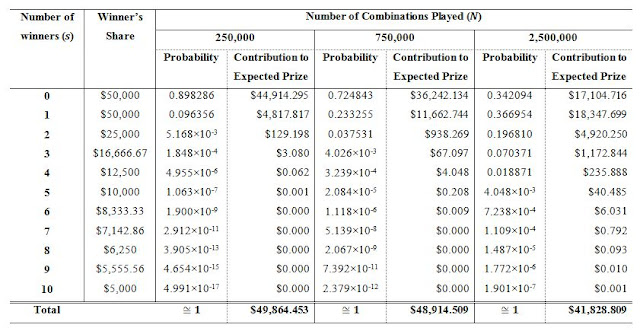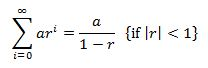## Monday 24 August 2015

### Gambling and Expected Value: Ontario 49 (OLG)

In this post on Gambling and Expected Value, we look at the "Ontario 49" lottery offered by the OLG.
Click here to find similar posts on other lotteries and games of chance.

### Ontario 49 (OLG)

Ontario 49 is a lottery offered by the Ontario Lottery and Gaming Corporation (OLG).

To play Ontario 49, the player selects a combination of 6 numbers chosen from 1 to 49. Numbers cannot be repeated and the order is not important. The player also has the option of having the computer generate a random selection instead (known as "Quick Pick" in lottery vernacular). The six winning numbers are drawn at random, along with a seventh "bonus number". The bonus number is only important for the second or sixth prizes. Second prize is awarded to tickets matching 5 out of 6 numbers plus the bonus number. Sixth prize is awarded to tickets matching 2 out of 6 numbers plus the bonus number. Winnings are determined by how many of the numbers are matched. The minimum wager in Ontario 49 is $1 and additional plays are bought in$1 increments. A $1 wager buys 1 play. ### Probabilities and Prizes There are 7 different ways to win a prize playing Ontario 49, ranging from a free ticket in a future draw to$2,000,000. The first and second prizes are capped to a total of $2,000,000 and$50,000 respectively, meaning that if there are multiple winners, the prize gets split between them.

There are 13,983,816 unique combinations of six numbers that can be chosen from the numbers 1 to 49. That number of combinations of items you can choose from a set without repetition is calculated using the following formula:where k is the number of items chosen, n is the total number of item to choose from, and ! denotes the factorial function. In this case, n = 49 and k = 6.

The probability of matching some number of the winning 6 can be calculated from the hypergeometric distribution. The formula looks like this:Equation 1 (general lottery prize probability formula)

where p is the probability of winning, n is the size of the set of numbers the winners are drawn from, k is the size of a combination, and r is the number of matching numbers required to win the prize. For example, to calculate the probability of matching three numbers in Ontario 49, r = 3, k = 6, and n = 49. Plugging in the numbers gives the probability of approximately 1.765%, or about 1 in 56.7.

The second prize adds a complication because of the bonus number. The formula to calculate the probability of matching r out of k winning numbers plus a bonus number (also drawn from the pool of n numbers) is a slight modification of Equation 1:Equation 2 (general lottery with bonus number prize probability formula)

Here is a table summarizing Ontario 49's payouts and their probabilities:

Since matching 5 out of 6 numbers has two different prizes depending on whether or not you match the bonus, the probability has to be adjusted for overcounting. The probability of matching 6 of 6, 4 of 6, or 3 of 6 numbers is calculated from Equation 1. The probability of matching 5 of 6 plus the bonus or 2 out of 6 plus the bonus is calculated from Equation 2. The remaining prizes, matching 5 of 6 but not the bonus, or 2 out of 6 but not the bonus, is calculated from Equation 1 minus Equation 2.

The last thing we need is the probability that your wager is just money thrown away. That's 100% minus the total of probabilities of winning the prizes in the table above, which works out to exactly 70,662,652,981/83,230,673,988 (about 84.9%). This means that an Ontario 49 number combination has about 15.1% chance of not losing. However, keep in mind most of those "winners" are just getting a free play in a future draw, which as you'll see in the expected value section, is almost worthless.

### Expected Value

The precise expected value is a little tricky because the payout on the top two prizes depends on how many winners there were, and the value of a free play is actually significantly less than the cost of the play. Let's first start by calculating the contribution to the expected value coming from the guaranteed prize amounts. Each contribution is simply the net gain (prize minus wager) multiplied by the probability.

Next, lets calculate how much the losing tickets contribute to the expected value. Again, it's the net gain multiplied by the probability, or (-$1) * (70,662,652,981/83,230,673,988). That's about -$0.849. At this point, the expected value of a ticket, ignoring the top two prizes and the free tickets, is about -$0.609. Next we have to figure out how much the top two prizes contribute to expected value. That depends on how many winners there are. We don't know how many winners there are, but if we know how many combinations are played, we can estimate how likely there are to be 0, 1, 2, etc. winners. This is calculated using the binomial distribution. If the probability of winning the prize is p, the probability of not winning the prize is (1-p), and N combinations are played, then probability of there being s winners of the shared pot is: What do we need this for? Well, because the prize depends on the number of winners and the number of winners is a probabilistic function of the number of tickets sold. Therefore, we need to estimate an expected value of the prize. I've estimated that the number of combinations played in each Ontario 49 draw is on the order of 750,000. Accordingly, I've estimated the expected top prize based on there being up to 7 winners (more than 7 winners is too unlikely to be worth consideration). I've also checked N = 250,000 and N = 2,500,000 to show that the final result is not that sensitive to the accuracy of my estimate of N.Probability of s winners of the top prize in a draw where N random combinations are played. Expected prize amount based on N combinations played. The expected value of the top prize is$1,998,603.85 if 750,000 combinations are played in each draw. So for the top prize, the effect of prize sharing is pretty small, assuming my estimate of 750,000 combinations played per draw is reasonable. As you can see from the table, even if I was off by a factor of more than three and 2,500,000 combinations are played, the expected prize is still within 0.8% of the single-winner prize amount.

Working through the same process for the second prize, this time considering up to 10 winners because the likelihood of multiple second prize winners is higher, we get the following table:Probability of s winners of the second prize in a draw where N random combinations are played.Expected prize amount estimated based on N combinations played.

You can see from the difference between N = 750,000 and N = 2,500,000 that the expected payout for the second prize is little more sensitive to the accuracy of my estimate of N. But it is not excessively sensitive, so our estimate is probably still pretty close to correct.

Finally, we need to estimate the value of a free ticket. Start by taking the expected value of free ticket. It's the sum of the products of each prize and the likelihood of winning. Let's consider only the monetary prizes first.Expected value of a free ticket, ignoring the value of additional free tickets.

Summing up the expected values of the prizes on a free ticket works out to a little under 43.5 cents. But a free ticket has roughly 12% probability of win another free ticket, and that ticket could win a monetary prize, or it could win yet another free ticket, and so on. What we have here is an infinite series that can be expressed by the equation belowThe infinite summation to calculate the equivalent prize value of a free Ontario 49 ticket.
We could sum the first few terms until it converges, but it this case we have a very simple closed-form solution, shown below:Solution to the sum of an infinite power series.
Therefore,
So winning a free ticket is equivalent to receiving a cash prize of about 49.4 cents.

We can also use a similar infinite sum to calculate the probability of getting any money back on an Ontario 49 ticket. The value of a becomes the sum of the probabilities of winning the monetary prizes and r is the probability of winning a free ticket.

Thus, after accounting for the possibility of winning money on a free ticket, the chance of winning money on an Ontario 49 play is still only about 3.52% (i.e. about 1 in 28.4).

Putting everything together now, the expected value of a single Ontario 49 play is

This means that, on average, for every dollar you spend playing Ontario 49, the OLG keeps about 50.6 cents for themselves.

From our analysis we can draw the following conclusions:

1. Ontario 49 has a house edge of about 50.6%, which is slightly worse than a 50/50 draw. In other words, on average, OLG keeps 50.6 cents of every dollar spent playing Ontario 49.
2. The OLG website is misleading in stating that your odds of winning are 1 in 6.6. There's only 3.52%, or 1 in 28.4, chance that you're going to win any money.

1.This is such a great resource that you are providing and you give it away for free. I love seeing websites that understand the value of providing a quality resource for free. It is the old what goes around comes around routine. agen judi

2.I'm glad I found this web site, I couldn't find any knowledge on this matter prior to.Also operate a site and if you are ever interested in doing some visitor writing for me if possible feel free to let me know, im always look for people to check out my web site. Click…

3.Buy a fake GED diploma, certificate or transcript that looks real at a great ... best fake GED maker and THE source for the most realistic fake GED
passportsservices247@gmail.com

Telegram=@Passportsservicese

https://t.me/joinchat/NNLD9_LDZH9mMjY0

4.Good day everybody, This is my testimony on how i won $4,200,201 million I want to use this opportunity to thank Great Priest Salami for helping me to win the lottery of$4,200,201 million dollars on Mega millions lottery ticket. I have been playing the lottery for the past 10 years now and I have never won. Ever since then I have not been able to win and I was so upset and I needed help to win the lottery. So I decided to go online and search for help, there i saw so many good testimonies about this man called Great Priest Salami of how he has cast lucky spell lotto for people to win the lottery. I contacted him also and told him I want to win a lottery, he cast a spell for me which I use and I played and won \$4,200,201 million dollars. I am so grateful to this man, just in-case you also need him to help you win, you can contact him through his email: purenaturalhealer@gmail.com WhatsApp number +2348143757229

5.Nagaland State Lottery Sambad Today 6:00 PM Result – June 24 2022